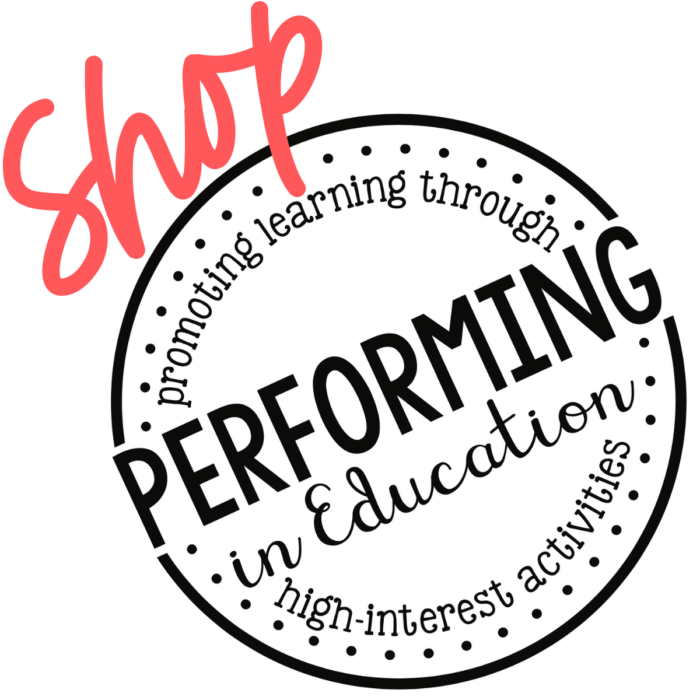\$5.00

# Math Project-Based Learning: Decimal Place Value | 5th Grade Math

\$5.00

## Description

This 5-day project-based math resource applies math concepts in a real-world scenario to teach students about decimal place value. In this 5-day unit, students will use concepts of decimal place value to shop for school supplies. This decimal place value math project is engaging and authentic. Plus, it’s available as both a digital and print resource.

This project-based math resource covers concepts of place value with decimals and is aligned with the following 5th Grade Math Common Core State Standards:

CCSS.MATH.CONTENT.5.NBT.A.3
Read, write, and compare decimals to thousandths.

CCSS.MATH.CONTENT.5.NBT.A.3.A
Read and write decimals to thousandths using base-ten numerals, number names, and expanded form, e.g., 347.392 = 3 √ó 100 + 4 √ó 10 + 7 √ó 1 + 3 √ó (1/10) + 9 √ó (1/100) + 2 √ó (1/1000).

CCSS.MATH.CONTENT.5.NBT.A.3.B
Compare two decimals to thousandths based on meanings of the digits in each place, using >, =, and < symbols to record the results of comparisons.

CCSS.MATH.CONTENT.5.NBT.A.4
Use place value understanding to round decimals to any place.

This resource is available in both Printable & Digital Google Slides versions.

What’s included in this resource:

• Background Knowledge: Using a KWL, students will build background knowledge about shopping for school supplies.
• Place Value Concepts: Using the included instructional pages, students will review place value concepts such as standard form and word form.
• Choose School Supplies: Students will make a list of ten school supplies to purchase.
• Expanded Form Practice: Using concepts of decimal place value, students will learn how to write a decimal in expanded notation.
• Compare and Order Numbers: Using the included instructional pages, students will learn how to compare and order decimal numbers.
• Make a Shopping List: Students will make an ordered list of the cost of the school supplies they wish to purchase, using place value strategies.
• Round Decimals: Using the included instructional pages, students will learn how to round decimal numbers.
• Estimate Total Cost: Using concepts of decimal place value, students will round the cost of each school supply to estimate the total cost.
• Compare Prices: Using concepts of decimal place value, students will compare the cost of each school supply at two different stores.
• Recalculate Cost: Students will choose the best price for each school supply and make a final list.
• Purchase Supplies: Using concepts of decimal place value, students will determine how the total price of their supplies compares to the estimated cost, and determine how many bills and coins they will need to make their purchase.
• Wrap Up: Students will decorate a school notebook, write up their final back to school shopping plan, and explain their decisions.

***************************************************************************

## Reviews

There are no reviews yet.

Only logged in customers who have purchased this product may leave a review.

Select